Home > CCA2 > Chapter 9 > Lesson 9.1.3 > Problem9-44

9-44.
1. Change the angle measurements below from radians to degrees or from degrees to radians. Homework Help ✎

1. 144°

2. 300°

3.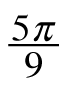4.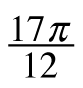5.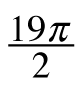6. 220°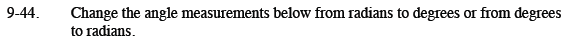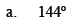$\frac{144^{\circ}}{180^{\circ}} = \frac{x}{\pi}$

$\frac{144^{\circ}}{180^{\circ}} = \frac{x}{\pi} \; \text{so} \; \frac{4}{5}\pi = x$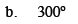$270^{\circ}+30^{\circ}$

$\frac{3}{2} \pi + \frac{1}{6}\pi = \frac{10}{6}\pi = \frac{5}{3}\pi$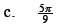$\pi=180^{\circ}$

$\frac{5 \cdot 180^{\circ}}{9} = 5 \cdot 20^{\circ}= 100^{\circ}$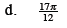Follow a process like (c) above.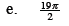Follow a process like (c) above.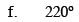Follow a process like (a) above.